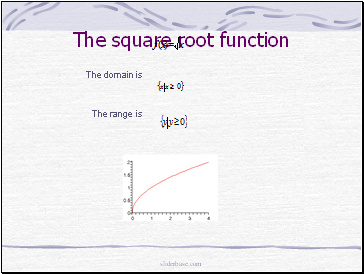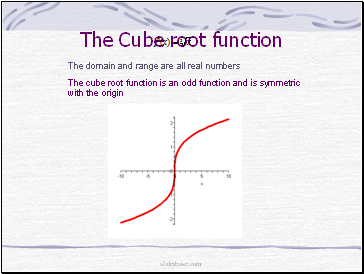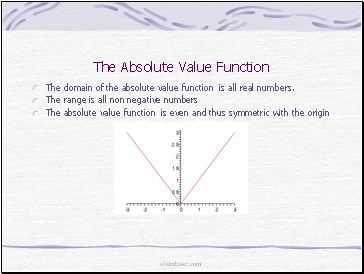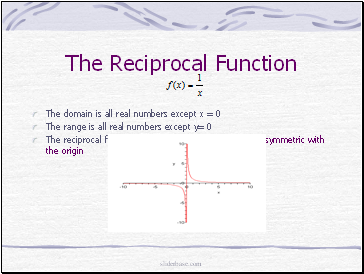# Properties of FunctionsPage 2

#### WATCH ALL SLIDES

The domain and the range are all real numbers

The x and y intercepts are at (0,0)

The cube function is an odd function and thus is symmetric with the origin

sliderbase.com

Slide 9## The square root function

The domain is

The range is

sliderbase.com

Slide 10## The Cube root function

The domain and range are all real numbers

The cube root function is an odd function and is symmetric with the origin

sliderbase.com

Slide 11## The Absolute Value Function

The domain of the absolute value function is all real numbers.

The range is all non negative numbers

The absolute value function is even and thus symmetric with the origin

sliderbase.com

Slide 12## The Reciprocal Function

The domain is all real numbers except x = 0

The range is all real numbers except y= 0

The reciprocal function is an odd function and is thus symmetric with the origin

sliderbase.com

Go to page:
1  2